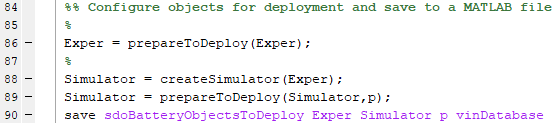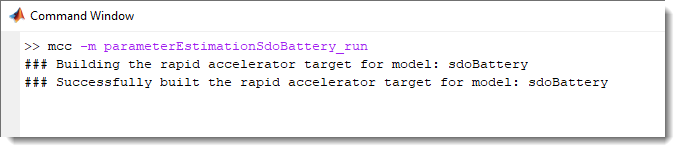Main Content

# Deployed Application of Parameter Estimation

This example shows how to monitor the condition of an electric vehicle battery in the field, with a deployed version of parameter estimation in Simulink® Design Optimization™, together with Simulink Compiler™.

### Battery Monitoring

Batteries in electric vehicles are expensive to replace and need to be monitored and maintained carefully, to ensure they function well for their intended lifetime. In this example, an electric car is driven to work and back on a daily commute. At home, the car is plugged in to a smart charger that monitors both the current and the battery voltage. The charger analyzes the battery data to estimate the battery parameters, using a deployed version of parameter estimation in Simulink Design Optimization, together with Simulink Compiler. The charger relays these parameters to the car manufacturer through an Internet of Things (IoT) connection, so that the manufacturer can monitor the battery health over time.

### Battery Model

This example estimates parameters of a simple, rechargeable battery model, `sdoBattery`. The input to `sdoBattery` is the battery current, and the model output is the battery terminal voltage which is computed from the battery state of charge.

The battery model is based on the equation:

`$\mathit{E}=\left(1-\mathrm{Loss}\right)\mathit{V}-\mathit{K}{\mathit{Q}}_{\mathrm{max}}\frac{1-\mathit{s}}{\mathit{s}}$`

where:

• $\mathit{E}$ is the battery terminal voltage in Volts.

• $\mathit{V}$ is the battery constant voltage in Volts.

• $\mathit{K}$ is the battery polarization resistance in Ohms.

• ${\mathit{Q}}_{\mathrm{max}}$ is the maximum battery capacity in Ampere-hours. ${\mathit{Q}}_{0}$ is the battery initial state of charge in Ampere-hours.

• $\mathit{s}$ is the battery charge state, with 1 being fully charged and 0 discharged. The battery state of charge is computed from the integral of the battery current with a positive current indicating discharge and a negative current indicating charging.

• $\mathrm{Loss}$ is the voltage drop when charging, expressed as a fraction of the battery constant voltage.

Use the following command to open the model.

`open_system('sdoBattery')`

### Battery Characteristics

The following battery characteristics are known:

• Voltage,$\mathit{V}=$ 400V

• Loss factor, $\mathrm{Loss}$ = 0.012

• Resistance, $\mathit{K}=$ 0.32 Ohms.

${\mathit{Q}}_{\mathrm{max}}$ is known to be 250 Ampere-hours (100 kWh) when the battery is new. As the battery ages, ${\mathit{Q}}_{\mathrm{max}}$ is expected to decrease, and this is monitored to track the health of the battery. The initial state of charge ${\mathit{Q}}_{0}$ and the new battery capacity ${\mathit{Q}}_{\mathrm{max}}$ need to be estimated.

### Steps for Deployed Parameter Estimation

There are two main steps to run parameter estimation in deployed mode:

1. Make a setup file, to set up parameter estimation objects for use in deployed mode

2. Make a run file, which is a MATLAB function for parameter estimation that can be compiled and run in deployed mode

It is recommended to create the setup and run files by starting with MATLAB code generated from the Parameter Estimator. Copy, split, and modify the generated code to make the setup and run files as demonstrated in the following section.

### Parameter Estimation in Non-Deployed Mode

First generate MATLAB code to estimate ${\mathit{Q}}_{0}$ and ${\mathit{Q}}_{\mathrm{max}}$ in non-deployed mode. Use the following commands to load the pre-configured estimation session:

```load sdoBattery_spesession_forDeployment spetool(SDOSessionData) ```

This step loads and plots the experiment with measured voltage and current data and configures the Parameter Estimator to estimate ${\mathit{Q}}_{0}$ and ${\mathit{Q}}_{\mathrm{max}}$.

Navigate to the Estimate button in the toolstrip and from the dropdown list, select Generate MATLAB Function (see Generate MATLAB Code for Parameter Estimation Problems (GUI)). This step generates a MATLAB function which is added to the MATLAB editor, and a MAT-file `parameterEstimation_sdoBattery_Data.mat`. The generated code is available for you in file `parameterEstimationSdoBattery.m`. You can use the generated code to estimate parameters in non-deployed mode.

It is recommended to start with this generated code and copy, split and modify the code to create the setup and run files described in the following sections.

### Setup-File for Deployed Parameter Estimation

To estimate parameters in deployed mode, the code for non-deployed parameter estimation can be split into a setup file to use in non-deployed mode, and a run file to use in deployed mode. The setup file is available as `parameterEstimationSdoBattery_setup.m` and the main parts are:

1. Define parameters

2. Define experiments

3. Prepare for deployment and save

#### Define Parameters

Parameters are defined in `parameterEstimationSdoBattery_setup.m` in the same way as the generated MATLAB code, `parameterEstimationSdoBattery.m`. Use the `sdo.getParameterFromModel` command to create a parameter object, containing fields for parameter value, minimum and maximum, and a field ("Free") indicating whether the parameter will be tuned during estimation.

In this example, parameter information is also stored in a database in which cars are identified by a code akin to a pseudo vehicle identification number (VIN). The car manufacturer can use this to monitor the health of the battery over time. The `parameterEstimationSdoBattery_setup.m` file uses the VIN database to update battery parameter values. See the `parameterEstimationSdoBattery_setup.m` file for more details.

The initial database is loaded from the MATLAB file `sdoBatteryVinDatabase.mat` which has the VIN database stored in variable `vinDatabase`. This is a `containers.Map` object, and the VIN key `4DEF` is used to look up parameters for the battery in this example.

Run

```vinDatabase("4DEF") ```

to display the following table:#### Define Experiments

Experiments are defined in `parameterEstimationSdoBattery_setup.m` in the same way as the generated MATLAB code, `parameterEstimationSdoBattery.m`. Experiments have measured data and information about specific ports or signals in the model that are associated with the data.

#### Prepare for Deployment and Save

At the end of the `parameterEstimationSdoBattery_setup.m` file, define a simulator which can run the model and compare model output to measured data. Use the `prepareToDeploy` command to configure the experiments and simulator so they can be used in deployed mode. Save these prepared objects to a MAT-file.When running these steps on another model and preparing for deployment, you may be prompted to save the model to continue after running the setup function. Save the model to preserve logging settings that need to be in place for deployed mode.

The run file `parameterEstimationSdoBattery_run.m` uses the objects saved in `sdoBatteryObjectsToDeploy.mat` for parameter estimation in deployed mode.

### Run-File for Deployed Parameter Estimation

The run file is available as `parameterEstimationSdoBattery_run.m` and the main parts are:

1. Load preconfigured deployment objects

2. Update experiments and parameters

3. Run optimization

4. Update Parameter Database

#### Load Preconfigured Deployment Objects

The `parameterEstimationSdoBattery_run.m` needs a pragma so that the Simulink Compiler includes the model in the compiled code as follows:Load the preconfigured objects that were saved at the end of `parameterEstimationSdoBattery_setup.m` file as follows:#### Update Experiments and Parameters

The `parameterEstimationSdoBattery_run.m` file takes two input arguments:

• `dataFilename` $-$ a data file name for experiment data

• `vin` $-$ a vehicle identification number for parameter values

Read the data from the comma-separated-values (CSV) text file specified by `dataFilename`. Use the updateIOData command to update the deployed experiments with new input and output data (current and voltage data for this model). Since the data is from a CSV file, you do not need the `getData` function that is present in the generated MATLAB code, `parameterEstimationSdoBattery.m`.Use the VIN as a key to look up this car's battery parameters in the parameter database. Use the current value from the database to update the initial parameter values prior to running the new estimation. See the `parameterEstimationSdoBattery_run.m` file for more details.

#### Run Optimization

The next several steps in `parameterEstimationSdoBattery_run.m` are very similar to the code in `parameterEstimationSdoBattery.m` (for non-deployed estimation). Define a handle to the estimation objective function, specify optimization options, and use the `sdo.optimize` function. This step runs the model and compares model output to experiment data. Parameters are tuned to achieve a close match between the model and data.

The objective function is defined in the subfunction `sdoBattery_optFcn` which is also like the objective function in `parameterEstimationSdoBattery.m`. However, the name of the signal logging variable needs to be specified since it cannot be queried from the model in deployed mode.To determine the name of the variable ('`logsout`' in this case), query the model from MATLAB in non-deployed mode:

```get_param('sdoBattery','SignalLoggingName') ```

Alternatively, in Simulink use the Modeling tab in the toolstrip and click Model Settings. In the configuration dialog, select Data Import/Export and find the variable name in the `Signal` `logging` box.

#### Update Parameter Database

After calling `sdo.optimize` in the main function of `parameterEstimationSdoBattery_run.m`, update the VIN database. For each parameter that is estimated, copy the `CurrentValue` to the `PreviousValue` and then use the new parameter estimate to update the `CurrentValue`. See `parameterEstimationSdoBattery_run.m` for more details.### Running Parameter Estimation in Deployed Mode

Use the `mcc` command to compile the `parameterEstimationSdoBattery_run.m` function from either the MATLAB command window or the DOS or UNIX command prompt. You need to have MATLAB Runtime installed to complete the following steps. For more information, see Install and Configure MATLAB Runtime (MATLAB Compiler).Run parameter estimation in deployed mode.In MATLAB, run

```vinDatabase("4DEF") ```

to display the following result:### Tracking Battery Parameters over Time

The table below shows estimates of battery parameters ${\mathit{Q}}_{0}$ and ${\mathit{Q}}_{\mathrm{max}}$ over time. The file `sdoBattery_Data1.csv` contains data for the battery when it was new, `sdoBattery_Data2.csv` contains data for the battery when it was 1 year old and `sdoBattery_Data3.csv` contains data for the battery when it was 2 years old.Observe that there is degradation in battery capacity over time. There is a high rate of degradation in the first year after which the rate of degradation reduces. When the battery was new, the round-trip commute left the battery state of charge at 61% while after 2 years, the commute left the battery state of charge at 47%. If the state of charge falls below 40%, this condition reduces the number of times the battery can be recharged. By tracking battery parameters over time, the manufacturer can monitor the battery health, and determine if the car needs a new battery.

## SupportGet trial now